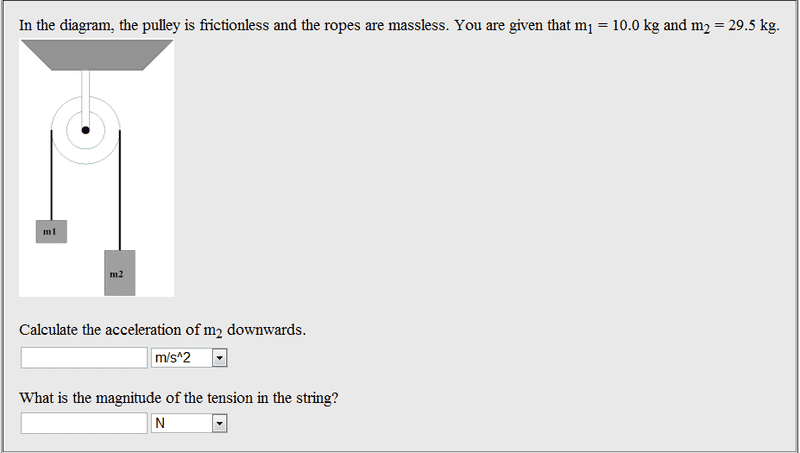# Pulley problem with frictionless ropes

Taz.

## Homework Statement

In the diagram, the pulley is frictionless and the ropes are massless. You are given that m1 = 10.0 kg and m2 = 29.5 kg.

Calculate the acceleration of m2 downwards.

What is the magnitude of the tension in the string?

sum of F= M*A

## The Attempt at a Solution

I tried doing a free body diagram and solving for A but I still can't find the tension...

LawrenceC
You should post a picture of the pulley layout to make the problem clear.

Taz.LawrenceC
OK, you have written Newton's Law. Draw a free body diagram of each weight calling the tension in the rope T. You should have two equations. Let's see them.

Taz.
m2:

force of tension going up
force of gravity going down

t1-w=ma

m1: same thing ...
t1-w=ma

LawrenceC
Let's use some subscripts because the masses/weights are different:

T - w1 = m1 * a

T - w2 = m2 * a

Looking at what you've written, you'll need to define what w1 and w2 are. Also, you have a single variable for acceleration. But the accelerations are in opposite directions so what must be done?

Taz.
So I have w1 and w2 and I have m1 and m2. Whats missing is T and A. Can I just solve for A or T ?

LawrenceC
So I have w1 and w2 and I have m1 and m2. Whats missing is T and A. Can I just solve for A or T ?

Yes you can but if you do you'll get an incorrect result. You have an error in one of your equations. Acceleration, like 'g', has a direction associated with it.

LawrenceC
Signing off for today.